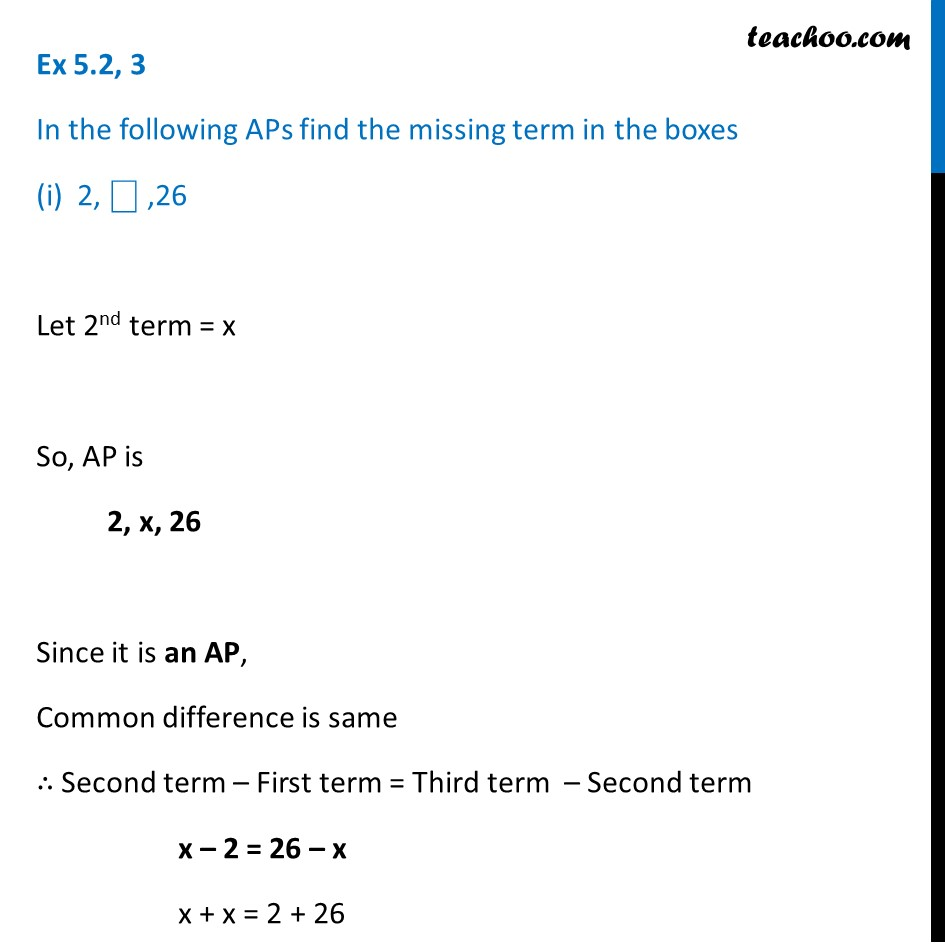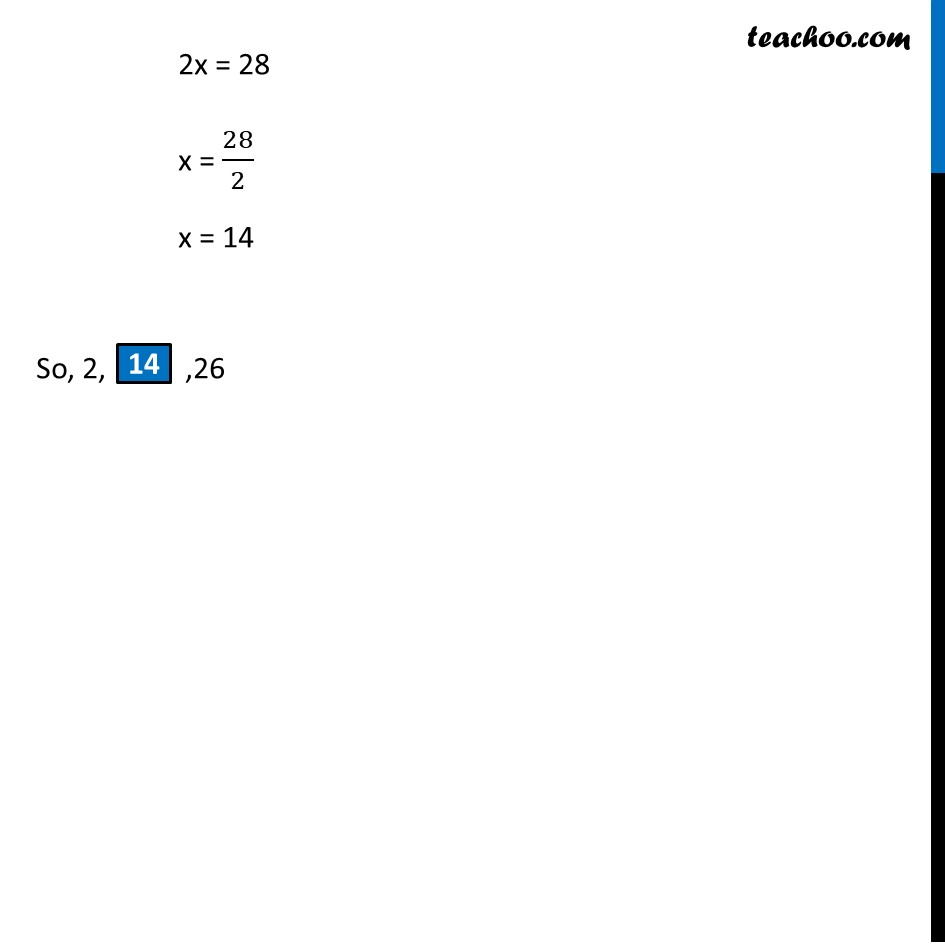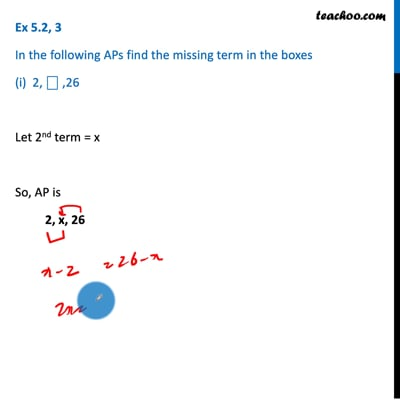Finding nth term

Chapter 5 Class 10 Arithmetic Progressions (Term 2)
Concept wiseThis video is only available for Teachoo black users

### Transcript

Ex 5.2, 3 In the following APs find the missing term in the boxes (i) 2, ⎕ ,26 Let 2nd term = x So, AP is 2, x, 26 Since it is an AP, Common difference is same ∴ Second term – First term = Third term – Second term x – 2 = 26 – x x + x = 2 + 26 2x = 28 x = 28/2 x = 14 So, 2, ,26